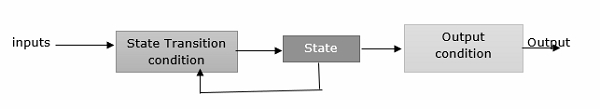# Explain the method for constructing Minimum Finite State Automata.

A finite state machine (FSM) which has a set of states and two functions called the next-state and output function.

The set of states correspond to all the possible combinations of the internal storage.

If there are n bits of storage, there are 2n possible states.

The next state function is a combinational logic function that given the inputs and the current state, determines the next state of the systemA Finite State Machine consists of the following −

• K states: S = {s1, s2, ... ,sk}, s1 is initial state
• N inputs: I = {h, i2, ... ,in}
• M outputs: O = {o1, o2, . ,om}

Next-state function T(S, I) is for mapping each current state and giving input to the next state. Output Function P(S) specifies the output.

The minimization of FSM means reducing the number of states from a given FA. Thus we get the FSM with redundant states after minimizing the FSM.

While minimising FSM we first find out which two states are equivalent. If two states are equivalent, then we can represent those two states by one representative state.

The two states q1, & q2 are equivalent if both δ(q1,x) and δ(q2,x) are final states or both of them are non-final states for all x ∈ Σ* (Σ* indicates any string of any length).

We can minimise the given FSM by finding equivalent states.

## Methods

The methods for constructing Minimum Finite State Automata are explained below −

Step 1 − Create a set S0 as S0={Q01, Q02}, where Q01 is the set of all final states and Q02 = Q-Q01 where Q is a set of all states in DFA.

Step 2 − Construct Sk+1 from Sk.

Let Qik be any subset in S0 . If q1 and q2 are in Qik, they are (k+1) equivalent provided δ(q1,a) and δ(q2,a) are K equivalent. Find out whether δ(q1,a) and δ(q2,a) are residing in the same equivalence class δ0.

Then, it is said that q1 and q2 are (k+1) equivalent. Thus, Qik is further divided into (k+1) equivalence classes

Step 3 − Repeat step 2 for every Qik in Sk and obtain all the elements of Sk+1

Step 4 − Construct Sn for n=1,2,3,…..until Sn=Sn+1

Step 5 − Then, replace all the equivalent states in one equivalence class by representative states.